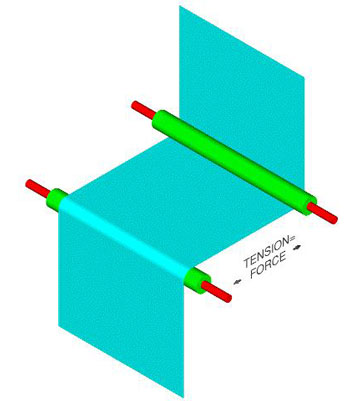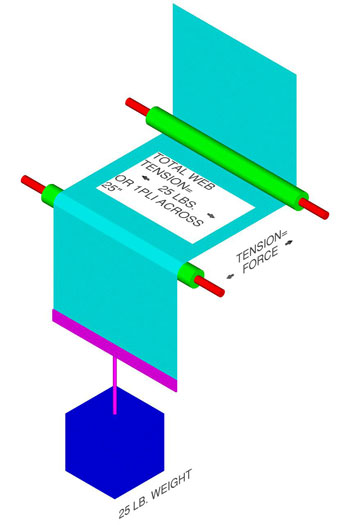# Tips

Home >>> Tech Tip

### What is web tension?

Tension is defined as the force applied to a continuous web of material in the machine direction.Typically, tension is measured in PLI (Pounds per Linear Inch) in the US. If you know PLI and you want to know total tension applied to the web, multiply PLI times the width of the material in inches. If you know total pounds of tension applied to the web and you want to know PLI, divide the total pounds of tension across the web by the width of the web in inches.

• PLI (Pounds per Linear Inch) = total pounds of tension / web width in inches
• Total pounds of tension = PLI X web width in inches
The tension applied to a web can be described as the webs tautness as if you hung a weight off the edge of the web. The tension on the web would be equal to the weight in pounds. PLI would be equal to the weight in pounds divided by the web width in inches.**DISCLAIMER - A great deal of time has been invested in the development of our weekly tech tips. To the best of our knowledge, they are accurate. It is up to the user to verify all results.

THE AUTHOR ASSUMES NO LIABILITY CONNECTED WITH THE USE OF THIS INFORMATION OR THE RESULTS OBTAINED FROM IT.